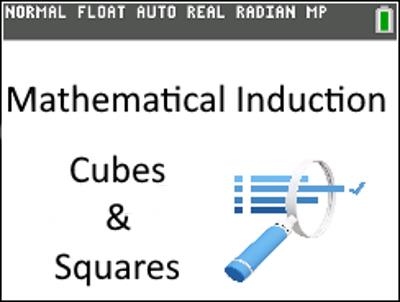Activities

•• AuthorAust Senior

30 Minutes

• Device
•TI-84 Plus CE

Part 3 of 3 - Induction Assessment 84Activity Overview

Part 3 of 3: Induction Assessment consists of a graded series of short answer questions - Exam style. The question follow a similar sequence to those in Parts 1 and 2. Students develop a formula for the sum of cubed numbers and compare it to the squared sum of whole numbers. Students use their calculator as applicable to answer questions which culminate in a proof by Mathematical Induction to show that their formula holds true.

Objectives

Part 1 of this 3 part series provided scaffolding to help students build formulas through visual, graphical and numerical methods and then prove their observations by process of Mathematical Induction. Part 2 removed much of the scaffolding but teachers interact with students providing feedback. Part 3 provides an assessment resource absent of scaffolding and support allowing students to independently work through a similar process.

Vocabulary

• Induction
• Sum
• Cumulative Sum
• Cubic